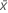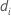Methods and formulas for Bootstrapping for 2-sample means

Select the method or formula of your choice.

Mean

A commonly used measure of the center of a batch of numbers. The mean is also called the average. It is the sum of all observations divided by the number of (nonmissing) observations.

Notation

TermDescription
xiith observation
Nnumber of nonmissing observations

Standard deviation (StDev)

The sample standard deviation provides a measure of the spread of your data. It is equal to the square root of the sample variance.

Formula

If the column contains x 1, x 2,..., x N, with mean, then the standard deviation of the sample is:

Notation

TermDescription
x i i th observationmean of the observations
N number of nonmissing observations

Variance

The variance measures how spread out the data are about their mean. The variance is equal to the standard deviation squared.

Notation

TermDescription
xiith observationmean of the observations
Nnumber of nonmissing observations

Sum

Notation

TermDescription
xi i th observation

Minimum

The smallest value in your data set.

Median

The sample median is in the middle of the data: at least half the observations are less than or equal to it, and at least half are greater than or equal to it.

Suppose you have a column that contains N values. To calculate the median, first order your data values from smallest to largest. If N is odd, the sample median is the value in the middle. If N is even, the sample median is the average of the two middle values.

For example, when N = 5 and you have data x1, x2, x3, x4, and x5, the median = x3.

When N = 6 and you have ordered data x1, x2, x3, x4, x5,and x6:

where x3 and x4 are the third and fourth observations.

Maximum

The largest value in your data set.

Average

Notation

TermDescriptiondifference in means of the ith resamples
B number of resamples
N number of observations for one group in the original sample

Standard deviation of the bootstrapping distribution

Notation

TermDescriptionmean of the differences of the resamples
B number of resamplesdifference in means of the ith resample

Confidence interval

Formula

Sort the difference in means of the resamples in increasing order. d1 is the lowest number, dB is the highest number.

Lower bound: dl where =Upper bound: du where =Note

For a one-sided case (only a lower bound or upper bound), use α instead of α/2.

When l or u are not integers, Minitab does a linear interpolation between the two numbers on either side of l or u. The formula is:

dy + z(dy+1 - dy)

For example, if l = 5.25, the lower bound equals d5 + .25(d6 - d5).

Minitab does not display the confidence interval whenor.

Notation

TermDescription
α 1- confidence level/100
B number of resamples
dy the yth difference when the data are sorted from least to greatest
y the truncated value of l or u
zl-y or u - y
By using this site you agree to the use of cookies for analytics and personalized content.  Read our policy